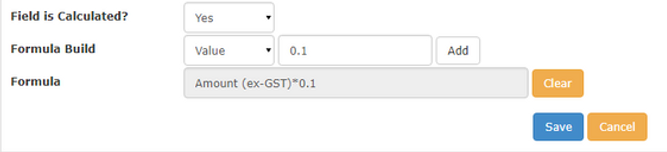top of page
Search

# ChilliDB Calculated Custom Fields

Updated: Oct 4, 2022

These fields enable you to build a Custom Field which can have its value calculated automatically based on a formula that you build. The following example is one of the ways to make use of the calculated fields. For this example we have used Base section in Notes module.

1. Create a custom field of type “Currency” called “Amount (ex-GST)”.2. Create a custom field called “GST” which is going to be our calculated field.3. The “Formula Build” list provides a grouping of elements which can be used to build a formula, including Field, Value and Operator. For our example, select “Field”. This will display another list of possible Custom Fields grouped under their Sections. It will only show you Fields which can be used in a formula, based on the Field Type you selected for this field. Since we chose to make our GST field a Currency, this will limit the available fields to numbers and currency fields.Select the “Amount” field and click the Add button to add that field to your formula box. This will keep building the Formula, as shown below.Now that we have referenced a Custom Field, we need to determine the operator we are applying in our formula. Select “Operator” from the Formula Build list, then select “*” in the next list, then click the Add button to put this into the formula box.As we are calculating GST, which logically is a calculation of \$ x 10%, we can represent the % portion as 0.1. Select “Value” from the Formula Build list, then enter “.1” in the following text box and click the Add button. This will build the formula as shown below.We have now completed our calculated field. Click the Save button to save the calculated custom field. Clear button will clear the whole Formula field and allow you to start again. Since we created this field against our Notes page, in the Base section of the form, find a choose Notes > Create from the ChilliDB menu.

Calculated fields work on the Save, so during an Add or Edit, your field will display “Calculated”. In the “Amount (ex-GST)” field, enter 1000 and click the Save button.After the save has completed, if you locate your GST field, you will see that it holds the calculated value.The following custom field types can also be calculated fields:

• Whole Number – Operators: Addition, subtraction, multiplication, brackets. Description: Returns a whole number from calculations using defined values or values located in a number field

• Decimal – Operators: Addition, subtraction, multiplication, brackets. Description: Returns a decimal number from calculations using defined values or values located in a number field

• Date – Operators: Subtraction Constants: Current Date Description: Date values can be subtracted from each other to calculate the difference in days between two dates

• Time – Operators: Subtraction, addition Description: A whole number can be added to, or subtracted from a time value. This adjusts the hours in the time value

• Date & Time – Operators: Subtraction Constants: Current Date Description: Date or date & time values can be subtracted from each other to calculate the difference in days and time between two dates

• Text – Operators: Addition Description: Can join text from lookup/multi-select lists, text fields or text defined in the formula using the addition operator.